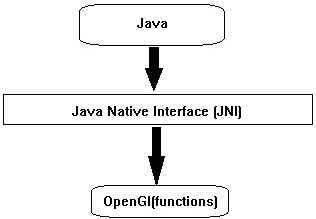# JOGL - Drawing Basics

OpenGL API has provided primitive methods for drawing basic graphical elements such as point, vertex, line etc. Using these methods, you can develop shapes such as triangle, polygon and circle. In both, 2D and 3D dimensions. This chapter teaches you how to draw a basic line using JOGL in a Java program.

## Drawing Objects

To access programs which are specific to a hardware and operating system platforms and where the libraries are written in other languages such as C and C++ (native applications), Java uses a programming framework called Java Native Interface (JNI). JOGL uses this interface internally to access OpenGL functions as shown in the following diagram.All the four methods of GLEventListener interface have the code (java JOGL methods) to call OpenGL functions internally. Naming of those JOGL methods is also similar to the naming conventions of OpenGL. If the function name in OpenGL is glBegin(), it is used as gl.glBegin().

Whenever the gl.glBegin() method of java JOGL is called, it internally invokes the glBegin() method of OpenGL. This is the reason for installing native library files on the user system at the time of installing JOGL.

## The Display() Method

This is an important method which holds the code for developing graphics. It requires the GLAutoDrawable interface object as its parameter.

The display() method initially gets OpenGL context using the object of GL interface (GL inherits GLBase interface which contains methods to generate all OpenGL context objects). Since this tutorial is about JOGL2, let us generate a GL2 object.

The following code snippet shows how to generate a GL2 Object −

```//Generating GL object
GL gl = drawable.getGL();
GL gl = drawable.getGL();

//Using this Getting the Gl2 Object
//this can be written in a single line like
final GL2 gl = drawable.getGL().getGL2();
```

Using the object of GL2 interface, one can access the members of this interface, which in turn provide access to OpenGL [1.0... 3.0] functions.

## Drawing a Line

GL2 interface contains a huge list of methods but here three main important methods are discussed namely glBegin(), glVertex(), and glEnd().

Sr.No. Methods and Description
1

glBegin()

This method starts the process of drawing a line. It takes predefined string integer “GL_LINES” as a parameter, which is inherited from GL interface.

2

glVertex3f()/glVertex2f()

This method creates the vertex and we have to pass coordinates as parameters 3f and 2f, which denote 3-dimensional floating point coordinates and 2-dimensional floating point coordinates respectively.

3

glEnd()

ends the line

Below given is the program to draws a basic line using JOGL −

```import javax.media.opengl.GL2;
import javax.media.opengl.GLAutoDrawable;
import javax.media.opengl.GLCapabilities;
import javax.media.opengl.GLEventListener;
import javax.media.opengl.GLProfile;
import javax.media.opengl.awt.GLCanvas;

import javax.swing.JFrame;

public class Line implements GLEventListener {

@Override
public void display(GLAutoDrawable drawable) {
final GL2 gl = drawable.getGL().getGL2();

gl.glBegin (GL2.GL_LINES);//static field
gl.glVertex3f(0.50f,-0.50f,0);
gl.glVertex3f(-0.50f,0.50f,0);
gl.glEnd();

}

@Override
public void dispose(GLAutoDrawable arg0) {
//method body
}

@Override
public void init(GLAutoDrawable arg0) {
// method body
}

public static void main(String[] args) {

//getting the capabilities object of GL2 profile
final GLProfile profile = GLProfile.get(GLProfile.GL2);
GLCapabilities capabilities = new GLCapabilities(profile);

// The canvas
final GLCanvas glcanvas = new GLCanvas(capabilities);
Line l = new Line();
glcanvas.setSize(400, 400);

//creating frame
final JFrame frame = new JFrame ("straight Line");